## Example Questions

### Example Question #138 : Trigonometry

A function with period P will repeat on intervals of length P, and these intervals are referred to as periods.

Find the period of the function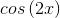.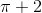Explanation:

For the function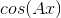the period is equal to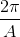or in this case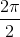which reduces to.

### Example Question #139 : Trigonometry

A function with period P will repeat on intervals of length P, and these intervals are referred to as periods.

Find the period of the function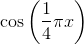.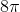Explanation:

For the functionthe period is equal toor in this case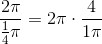which reduces to.

### Example Question #140 : Trigonometry

A function with period P will repeat on intervals of length P, and these intervals are referred to as periods.

Find the period of the function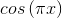.Explanation:

For the functionthe period is equal toor in this case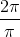which reduces to.

### Example Question #1 : How To Find The Period Of The Cosine

A function with periodwill repeat its solutions in intervals of length.

What is the period of the function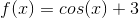?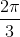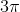Explanation:

For a trigonometric function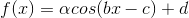, the periodis equal to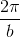. So, for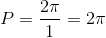.

### Example Question #2 : How To Find The Period Of The Cosine

A function with periodwill repeat its solutions in intervals of length.

What is the period of the function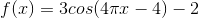?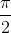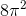Explanation:

For a trigonometric function, the periodis equal to. So, for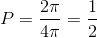.

### Example Question #3 : How To Find The Period Of The Cosine

A function with periodwill repeat its solutions in intervals of length.

What is the period of the function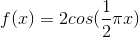?For a trigonometric function, the periodis equal to. So, for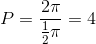.